# Chapter 2 Recursion The Mirrors Recursive Solutions Recursion

• Slides: 19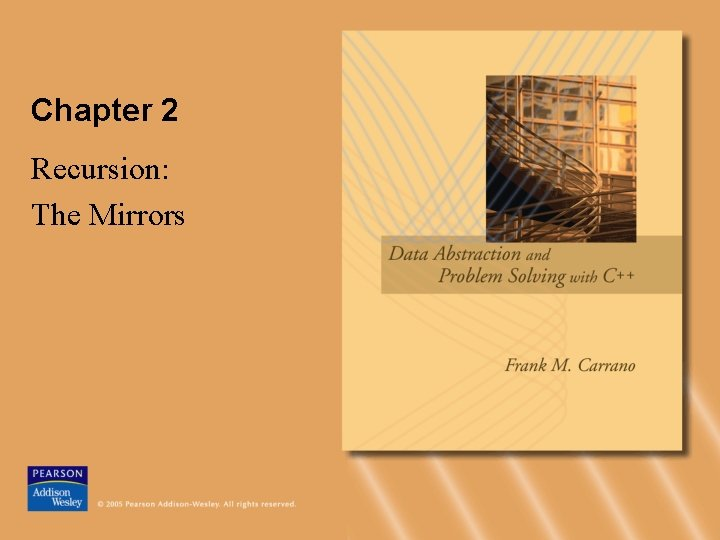Chapter 2 Recursion: The MirrorsRecursive Solutions • Recursion is an extremely powerful problemsolving technique – Breaks a problem in smaller identical problems – An alternative to iteration, which involves loops • A sequential search is iterative – Starts at the beginning of the collection – Looks at every item in the collection in order • A binary search is recursive – Repeatedly halves the collection and determines which half could contain the item – Uses a divide and conquer strategy © 2005 Pearson Addison-Wesley. All rights reserved 2Recursive Solutions • Facts about a recursive solution – A recursive method calls itself – Each recursive call solves an identical, but smaller, problem – A test for the base case enables the recursive calls to stop • Base case: a known case in a recursive definition – Eventually, one of the smaller problems must be the base case © 2005 Pearson Addison-Wesley. All rights reserved 3Recursive Solutions • Four questions for construction recursive solutions – How can you define the problem in terms of a smaller problem of the same type? – How does each recursive call diminish the size of the problem? – What instance of the problem can serve as the base case? – As the problem size diminishes, will you reach this base case? © 2005 Pearson Addison-Wesley. All rights reserved 4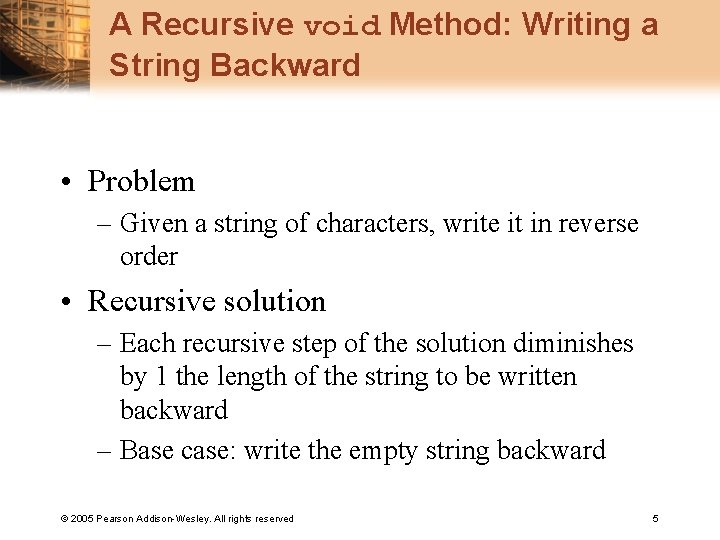A Recursive void Method: Writing a String Backward • Problem – Given a string of characters, write it in reverse order • Recursive solution – Each recursive step of the solution diminishes by 1 the length of the string to be written backward – Base case: write the empty string backward © 2005 Pearson Addison-Wesley. All rights reserved 5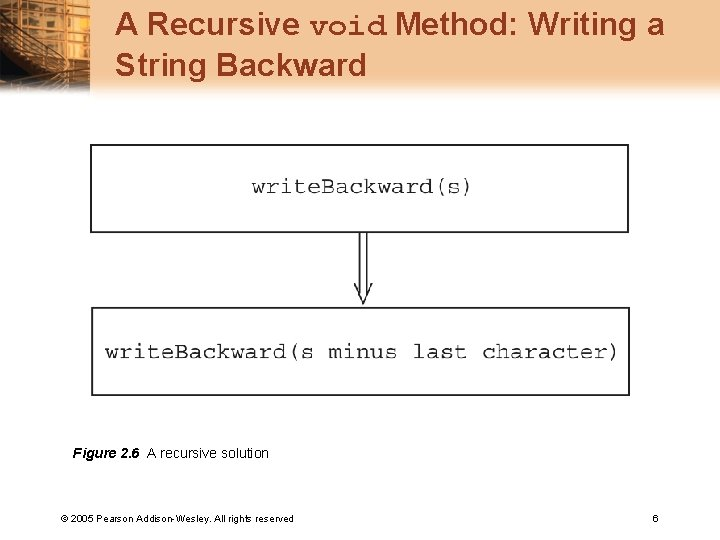Multiplying Rabbits (The Fibonacci Sequence) • “Facts” about rabbits – Rabbits never die – A rabbit reaches sexual maturity exactly two months after birth, that is, at the beginning of its third month of life – At the beginning of every month, each sexually mature male-female pair gives birth to exactly one male-female pair © 2005 Pearson Addison-Wesley. All rights reserved 7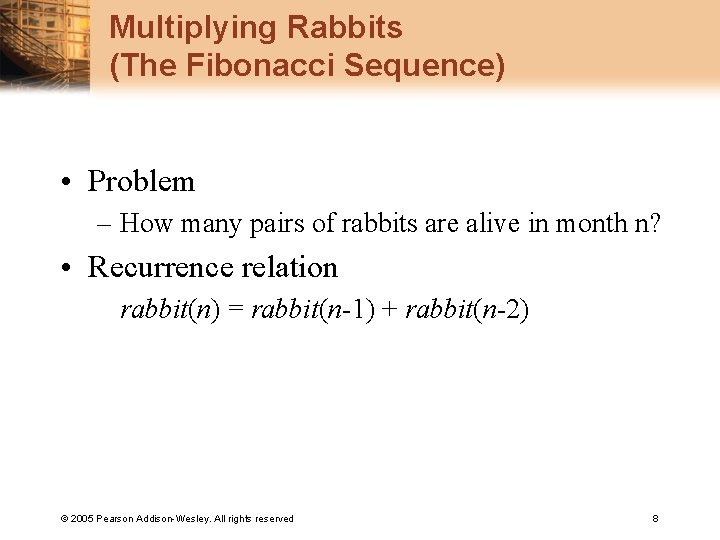Multiplying Rabbits (The Fibonacci Sequence) • Problem – How many pairs of rabbits are alive in month n? • Recurrence relation rabbit(n) = rabbit(n-1) + rabbit(n-2) © 2005 Pearson Addison-Wesley. All rights reserved 8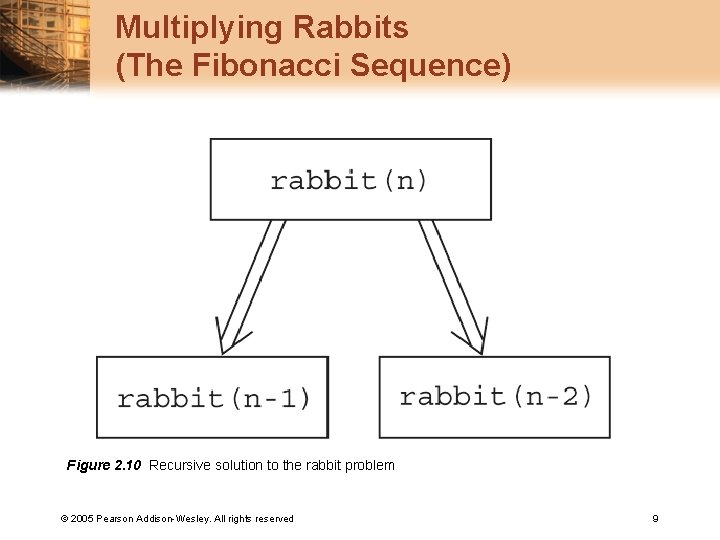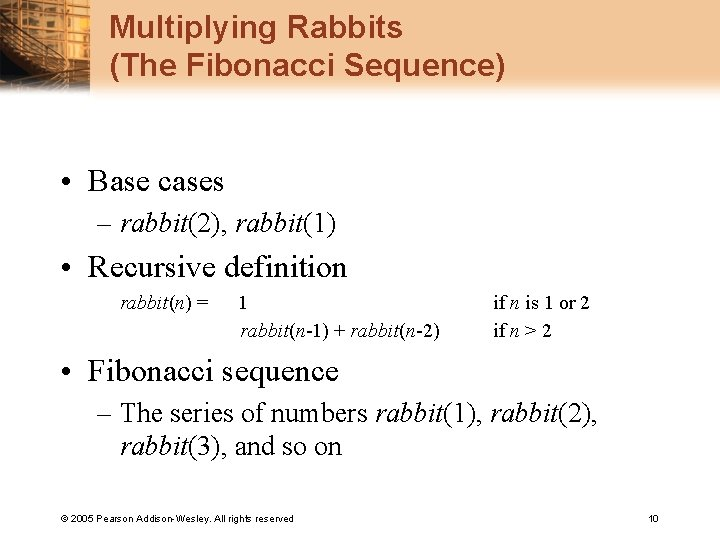Multiplying Rabbits (The Fibonacci Sequence) • Base cases – rabbit(2), rabbit(1) • Recursive definition rabbit(n) = 1 rabbit(n-1) + rabbit(n-2) if n is 1 or 2 if n > 2 • Fibonacci sequence – The series of numbers rabbit(1), rabbit(2), rabbit(3), and so on © 2005 Pearson Addison-Wesley. All rights reserved 10Implementation: Multiplying Rabbits (Recursive) int rabbit(int n){ // Precondition: n is a positive integer. // Postcondition: Returns the nth Fibonacci // number. if (n <= 2) return 1; else // n > 2, so n-1 > 0 and n-2 > 0 return rabbit(n-1) + rabbit(n-2); } © 2005 Pearson Addison-Wesley. All rights reserved 11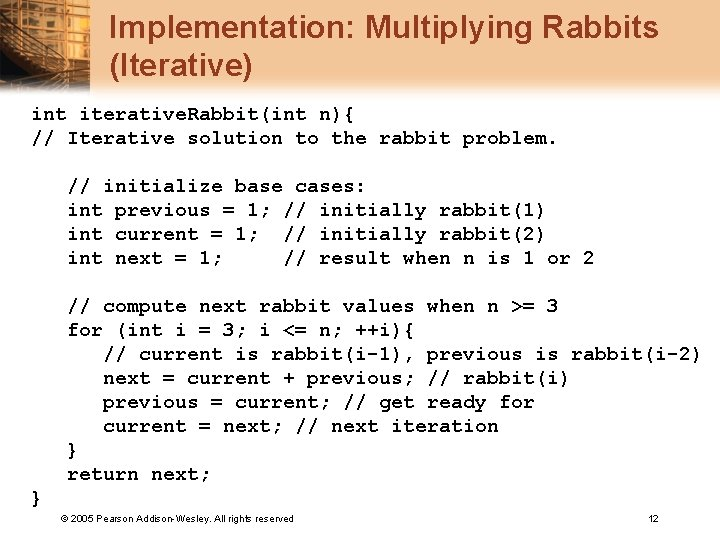Implementation: Multiplying Rabbits (Iterative) int iterative. Rabbit(int n){ // Iterative solution to the rabbit problem. // initialize base cases: int previous = 1; // initially rabbit(1) int current = 1; // initially rabbit(2) int next = 1; // result when n is 1 or 2 // compute next rabbit values when n >= 3 for (int i = 3; i <= n; ++i){ // current is rabbit(i-1), previous is rabbit(i-2) next = current + previous; // rabbit(i) previous = current; // get ready for current = next; // next iteration } return next; } © 2005 Pearson Addison-Wesley. All rights reserved 12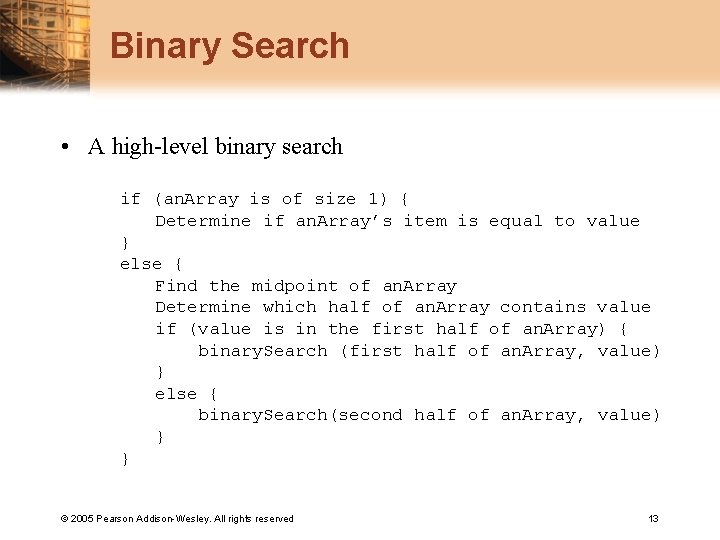Binary Search • A high-level binary search if (an. Array is of size 1) { Determine if an. Array’s item is equal to value } else { Find the midpoint of an. Array Determine which half of an. Array contains value if (value is in the first half of an. Array) { binary. Search (first half of an. Array, value) } else { binary. Search(second half of an. Array, value) } } © 2005 Pearson Addison-Wesley. All rights reserved 13Binary Search • Implementation issues: – How will you pass “half of an. Array” to the recursive calls to binary. Search? – How do you determine which half of the array contains value? – What should the base case(s) be? – How will binary. Search indicate the result of the search? © 2005 Pearson Addison-Wesley. All rights reserved 14Organizing Data: The Towers of Hanoi Figure a) The initial state; b) move n - 1 disks from A to C; c) move one disk from A to B; d) move n - 1 disks from C to B © 2005 Pearson Addison-Wesley. All rights reserved 15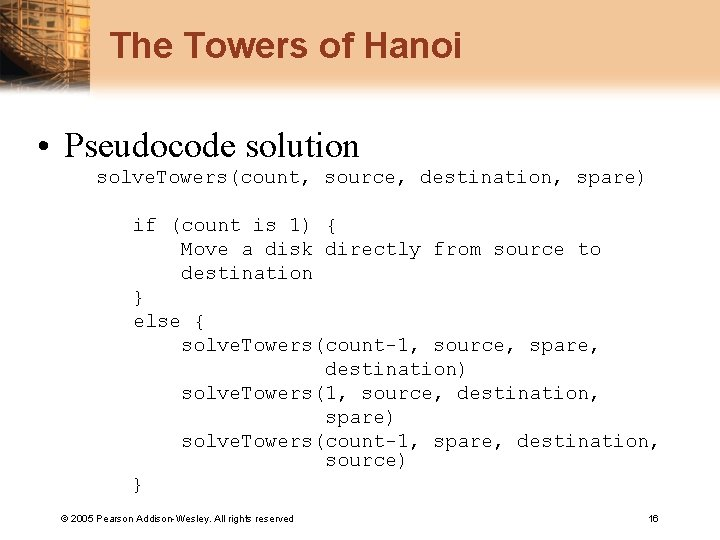The Towers of Hanoi • Pseudocode solution solve. Towers(count, source, destination, spare) if (count is 1) { Move a disk directly from source to destination } else { solve. Towers(count-1, source, spare, destination) solve. Towers(1, source, destination, spare) solve. Towers(count-1, spare, destination, source) } © 2005 Pearson Addison-Wesley. All rights reserved 16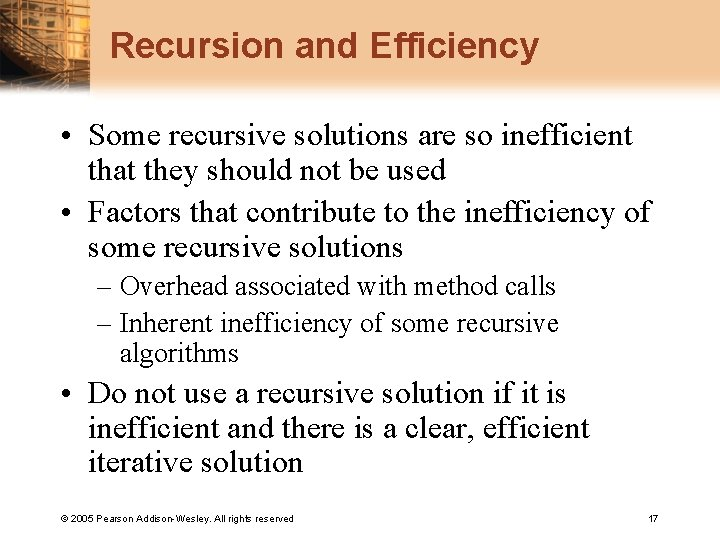Recursion and Efficiency • Some recursive solutions are so inefficient that they should not be used • Factors that contribute to the inefficiency of some recursive solutions – Overhead associated with method calls – Inherent inefficiency of some recursive algorithms • Do not use a recursive solution if it is inefficient and there is a clear, efficient iterative solution © 2005 Pearson Addison-Wesley. All rights reserved 17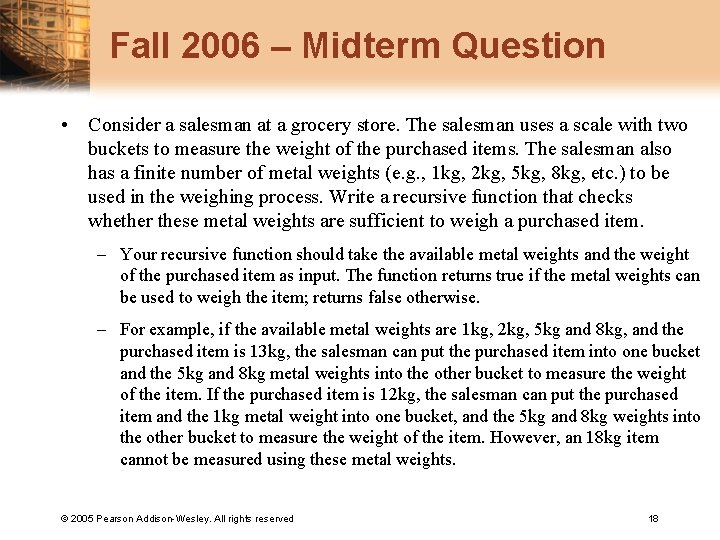Fall 2006 – Midterm Question • Consider a salesman at a grocery store. The salesman uses a scale with two buckets to measure the weight of the purchased items. The salesman also has a finite number of metal weights (e. g. , 1 kg, 2 kg, 5 kg, 8 kg, etc. ) to be used in the weighing process. Write a recursive function that checks whether these metal weights are sufficient to weigh a purchased item. – Your recursive function should take the available metal weights and the weight of the purchased item as input. The function returns true if the metal weights can be used to weigh the item; returns false otherwise. – For example, if the available metal weights are 1 kg, 2 kg, 5 kg and 8 kg, and the purchased item is 13 kg, the salesman can put the purchased item into one bucket and the 5 kg and 8 kg metal weights into the other bucket to measure the weight of the item. If the purchased item is 12 kg, the salesman can put the purchased item and the 1 kg metal weight into one bucket, and the 5 kg and 8 kg weights into the other bucket to measure the weight of the item. However, an 18 kg item cannot be measured using these metal weights. © 2005 Pearson Addison-Wesley. All rights reserved 18Fall 2006 – Midterm Question bool scale( const int *weights, const int size, const int item, const int i, const int sum ) { if ( sum == item ) return true; if ( i == size ) return false; bool result = false; result = scale( weights, size, item, i + 1, sum + weights[i]); if ( result == false ) result = scale( weights, size, item, i + 1, sum - weights[i]); if ( result == false ) result = scale( weights, size, item, i + 1, sum ); return result; } © 2005 Pearson Addison-Wesley. All rights reserved 19